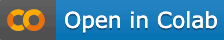# Next best action prediction### Import libraries​

``library(ChannelAttributionPro)``

``password="mypassword"``

``Data = read.csv("https://app.channelattribution.net/data/Data.csv",sep=",")print(Data)``

### Train the model​

``Params=next_best_action_train(Data, var_path="path", var_conv="total_conversions",                               var_null="total_null", row_sep="", cha_sep=">", password=password)print(Params)``

Params is a data.frame containing all the conversion rates estimated using a combination of Markov models. Params can be stored and used to predict next best action given a path.

### Predict next best action for a given path​

``new_path="beta > alpha"res=next_best_action(new_path,Params,sep=">",password=password)print(res)``

res contains the suggested next action, its conversion rate and the conversion rates for all the available actions.